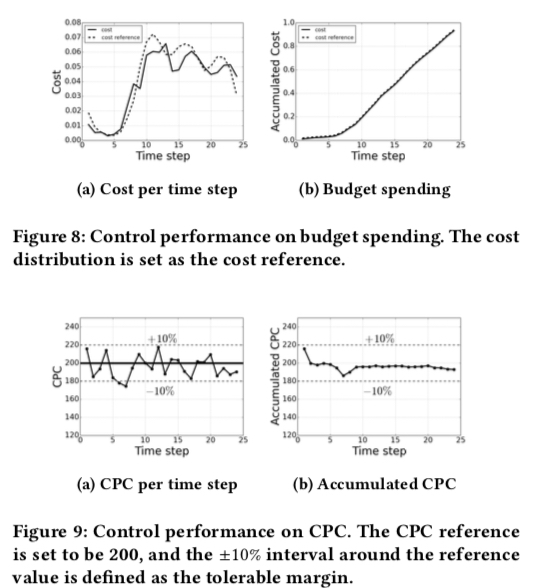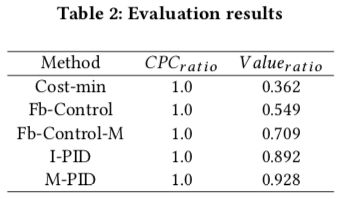(1) 为了满足广告主的各种诉求；如需要匀速投放时，即在一天内均匀地花完预算，这样就需要通过控制器来控制运算花费曲线趋势与大盘流量曲线的趋势保持一致；如需要保成本时，需要通过控制器在成本高了时压价, 低了时提价；需要跑量同时允许在成本有略微上涨时，可以在成本可控的情况下更激进一点出出价
(2) ctr/cvr 的预估不是完全准确的；常见的 ctr/cvr 高估时，容易导致超成本，因为这时候计算出来的 ecpm = bid×ctr×cvr 也相当于是高估了；其根本原因是在 ctr/cvr 预估中没有一个绝对的 ground truth, 我们能拿到的是点击/转化与否，但是要预估的则是点击/转化的概率

## 最优出价公式

paper 中要解决的场景是在保住点击成本和预算时，最大化广告主的在所有参竞价值(value)，因此这个优化问题可写成如下形式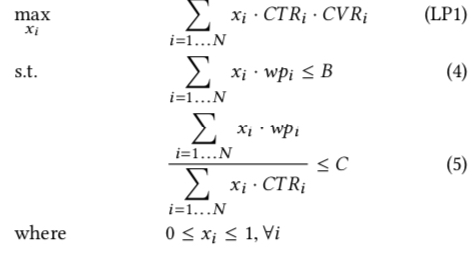• $N$, 广告计划的总的可参竞次数(opportunities)
• $x_i$, 第 i 次竞价获胜的概率
• $wp_i$, 第 i 次竞价的 winning price，即 bid price 要大于等于这个值才能获胜
• $B$, 计划的总预算
• $C$, 计划设置的点击成本

### paper 原始推导

paper 通过最优化中的对偶理论将上面的原问题(primal problem)转为对偶问题(dual problem)，如下图所示；对偶理论的详细描述可参考 最优化计算课程总结对偶理论这一节内容或 Duality Theory 这个讲义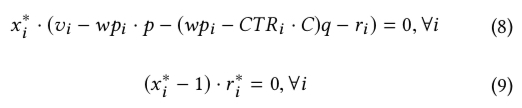$$bid^{*} = \frac{1}{p^*+q^*} × CTR_i × CVR_i + \frac{q^*}{p^*+q^*} × C × CTR_{i}\tag{6}$$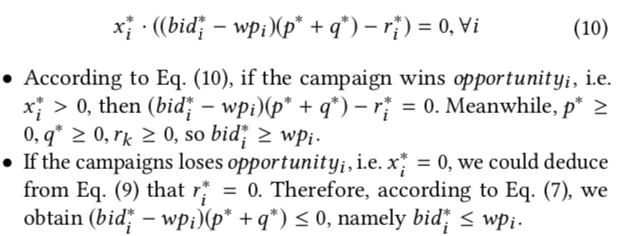### 另一种推导思路

$$L(x,p,q) = \sum_{i=1\dots N}x_iCTR_iCVR_i + p(\sum_{i=1\dots N}x_iwp_i-B) + q(\sum_{i=1\dots N}x_i(wp_i-CTR_iC))$$

$$\max_{p, q}\min_{x_i}L(x,p,q)$$

$$wp_i = \frac{1}{p+q} × CTR_{i} × CVR_{i} + \frac{q}{p+q} × C × CTR_{i}$$

### 小结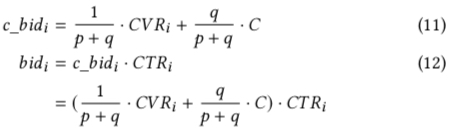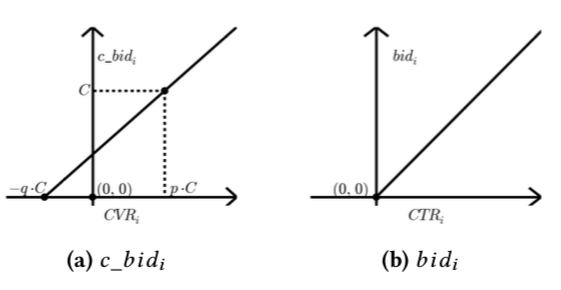## 调价策略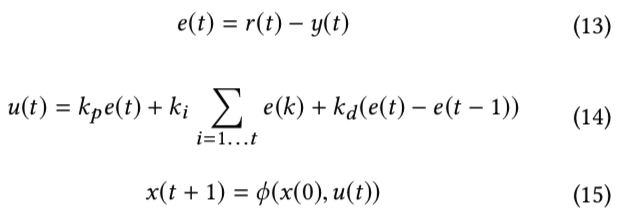PID 对于单变量的控制可以说是比较常用和有效的方法，但是我们的上面提到的问题是一个多变量控制问题，其中控制变量是 $p$ 和 $q$，控制目标是控制好预算花费并保点击成本; 对于这个问题，一个很直观的想法就是通过双 PID 分别进行独立调控，但是这些控制变量之间往往不是相互独立的，因此双 PID 不是最优的, 后面会详细描述这一点。

### 参数分析

• 出价的直线始终通过 (-qC, 0) 这个点
• 随着 $p$ 的减小，出价的直线的斜率逐渐增大，表示出价更高，同时消耗的 budget 也会更多;而随着 $p$ 增大导致的结果则是刚好相反
• 当 $p$ 取最小值即 0 时，表示没有 budget 的限制，出价公式退化为 $C×CTR+\frac{1}{q}CTR×CVR$, 公式第一项可以认为是只按照点击出价来保点击成本 $C$，第二项则是为了达到 $\max v=CTR×CVR$的目标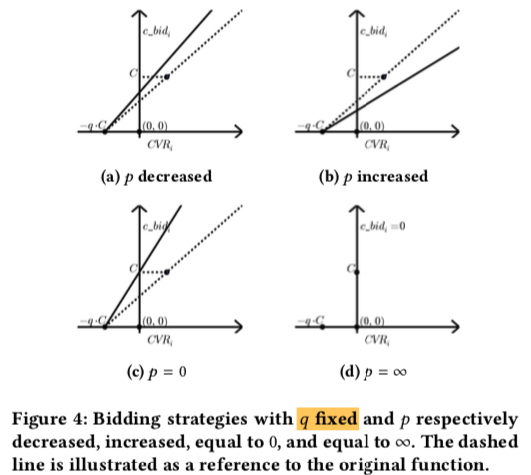• 出价的直线始终通过 (pC, C) 这个点
• 随着 $q$ 的减小，出价的直线的斜率逐渐增大，表示对于 CVR 比 pC 更高的流量出价更高，CVR 比 pC 更低的流量出价更低, 而随着 $q$ 增大导致的结果则是刚好相反
• 当 $q$ 取最小值即 0 时，表示没有点击成本的限制，此时的出价公式退化为 $\frac{1}{p}CTR×CVR$，代表出价成本的符号 $C$ 没有出现的出价公式中，总体表示要达到 $\max v=CTR×CVR$的目标，同时通过 $q$ 来控制预算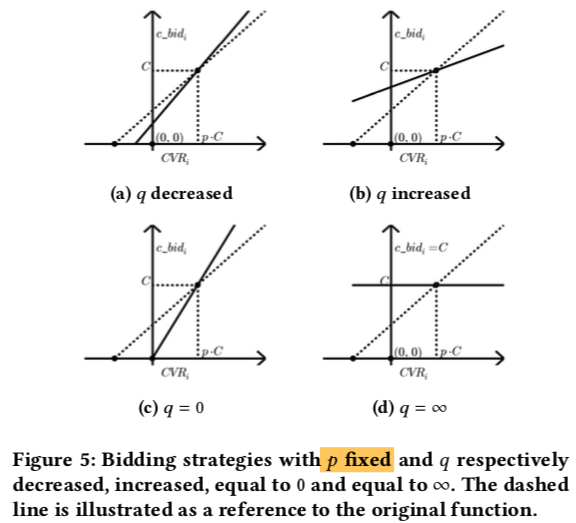### 控制器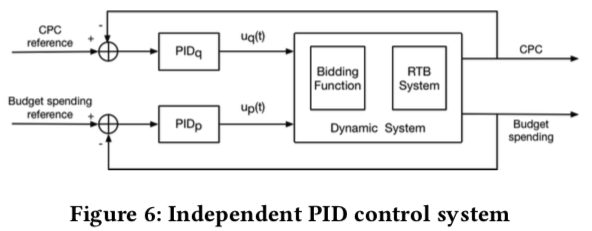Model Predict Control(MPC)中有关于这类问题的研究，但是 paper 中并不直接采用这个方法，因为 paper 中认为 “modelling the highly non-linear RTB environment is costly and even impratical“； 而是通过一个线性模型去拟合。笔者认为其可行的原因是调控往往会分为多个时间片，然后在每个时间片内进行调控，而在每个时间片内用直线去拟合，理论上只要把时间切得足够小，最终总体也能拟合出非线性的曲线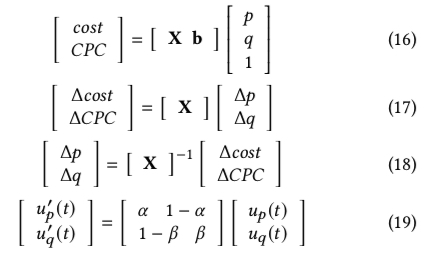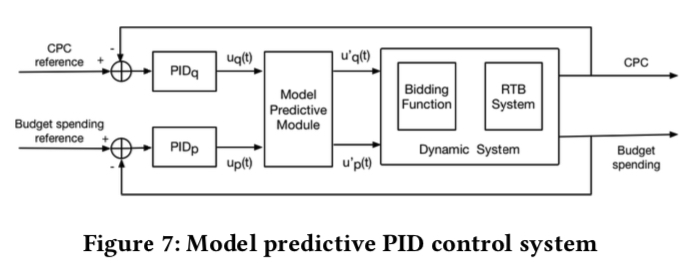## 实验设置与效果评估

### 基本设置

paper 中并没有进行在线实验，而是通过离线方式进行评估

• $CPC_{ratio}$: 表示保住点击成本的计划的比例
• $Value_{ratio}$：表示按最优出价公式重新投放时，每个计划获取的value与其理论最优的比值($\le 1$)

### 实现细节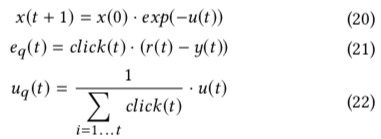(1) 基于训练数据集计算出最优的 $p$ 、$q$ 作为其初始值
(2) 在测试数据集上用上面的最优出价公式和调价策略进行模拟竞价
(3）当计划的 budget 消耗完或者所有的日志回放完成则终止

### 效果对比